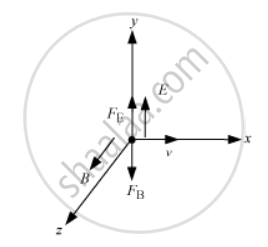Department of Pre-University Education, KarnatakaPUC Karnataka Science Class 12

# Find the Condition Under Which the Charged Particles Moving with Different Speeds in the Presence of Electric and Magnetic Field Vectors Can Be Used to Select Charged Particles of a Particular Speed. - Physics

Find the condition under which the charged particles moving with different speeds in the presence of electric and magnetic field vectors can be used to select charged particles of a particular speed.

#### Solution

Force in the presence of magnetic and electric field is given as

vecF=q(vecE+vecv xx vecB)

Consider that the electric and the magnetic field are perpendicular to each other and, also, perpendicular to the velocity of the particle.

vecE=Ehatj,vecB=Bhatk,vecB,vecv=vhati

Then we have :vec(F_E)=qvecE=qEhatj, vec(F_B)=q vecv xx vecB, =q(vhatixxBhatk)=-qBhatj

therefore vecF=q(E-vB)hatjIf we adjust the values of vecE and vecB such that magnitudes of the two forces are equal, then the total force on the charge will be zero and it will move in the fields undeflected. This happens when
qE = qvB

therefore v=E/B

The above condition can be used to select a charged particle of particular velocity from the charges moving with different speeds. Therefore, it is called velocity selector.

Concept: Motion in a Magnetic Field
Is there an error in this question or solution?